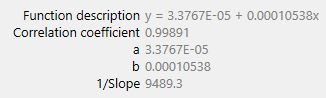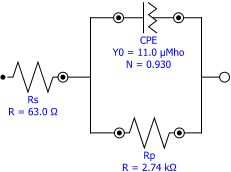You have been redirected to your local version of the requested page

In the previous application note, the procedure for estimating corrosion rates was outlined. The calculations were valid under the assumption that the corrosion reactions were under charge transfer control and that the mechanisms of the reactions were known. In real life, often, corrosion is a result of several reactions and it is not possible to determine a priori the reaction mechanism. In such cases, the polarization resistance can be used to determine the resistance of the metal under investigation against corrosion.

An electrode is polarized when its potential is forced away from its value at open circuit or corrosion potential. Polarization of an electrode causes current to flow due to electrochemical reactions at the electrode surface. The polarization resistance Rp is defined by the Equation 1:

Where ∆E (V) is the variation of the applied potential around the corrosion potential and ∆i (A) is the resulting polarization current.

The polarization resistance, Rp (Ω), behaves like a resistor and can be calculated by taking the inverse of the slope of the current potential curve at corrosion potential (OCP).

During the polarization of an electrode, the magnitude of the current is controlled by reaction kinetics and diffusion of reactants both towards and away from the electrode.

The Butler-Volmer relates the current 𝑖 with the overpotential 𝜂, Equation 2:

an-cor-003-2.png

The overpotential 𝜂(V) = EEcorr is defined as the difference between applied potential E and the corrosion potential Ecorr. The corrosion potential Ecorr is the open circuit potential of a corroding metal. The corrosion current icorr1 and the Tafel constants ba and bc can be measured from the experimental data.

For small overpotentials 𝜂, i.e. for potentials close to corrosion potential, the above equation can be reduced to:

Or, when the expression is rearranged:

If the Tafel slopes are known, the corrosion currents can be calculated from the polarization resistance using the above equations. If the Tafel slopes are not known (e.g., when corrosion mechanism is not known), Rp can still be used as a quantitative parameter to compare the corrosion resistance of metals under various conditions. A specimen with low Rp will corrode more easily than a specimen with a low Rp.

Linear Sweep Voltammetry (LSV)

In Figure 1, the results of a LSV experiment performed on an iron screw immersed in seawater are shown. The slope of the curve at Ecorr = −0.319 V can be calculated by performing a linear regression tangent to the data from -10 mV vs. Ecorr and +10 mV vs. Ecorr.

Figure 1. LSV data for the corrosion of an iron screw in seawaterFigure 2. The calculated regression line equation for the corrosion of an iron screw in seawater

The results of the regression are shown in Figure 2. The polarization resistance Rp is calculated from inverse of the slope (1/slope) and is found to be 9.489 kΩ.

Electrochemical Impedance SpectroscopyFigure 3. The equivalent circuit used to fit a semicircle in the Nyquist plot.

The polarization resistance can also be measured with electrochemical impedance spectroscopy (EIS). For simple systems where the Nyquist plot shows one semicircle, the equivalent circuit shown in Figure 3 can be used to estimate Rp.

In Figure 4, the Nyquist plot resulting from the corrosion of iron in sulfate solution is shown. The solid line represents the fit of the circuit shown to calculate the polarization resistance Rp.

Figure 4. Estimation of Rp for corrosion of iron in seawater using EIS